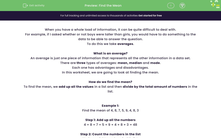# Find the Mean

In this worksheet, students will practise finding the mean from a small data set - discrete values only.Key stage:  KS 4

Year:  GCSE

GCSE Subjects:   Maths

GCSE Boards:   AQA, Eduqas, Pearson Edexcel, OCR,

Curriculum topic:   Statistics

Curriculum subtopic:   Statistics Analysing Data

Difficulty level:#### Worksheet Overview

When you have a whole load of information, it can be quite difficult to deal with.

For example, if I asked whether or not boys were taller than girls, you would have to do something to the data to be able to answer the question.

To do this we take averages.

What is an average?

An average is just one piece of information that represents all the other information in a data set.

There are three types of averages: mean, median and mode.

In this worksheet, we are going to look at finding the mean.

How do we find the mean?

To find the mean, we add up all the values in a list and then divide by the total amount of numbers in the list.

Example 1:

Find the mean of 4, 8, 7, 5, 9, 4, 8, 3

Step 1: Add up all the numbers

4 + 8 + 7 + 5 + 9 + 4 + 8 + 3 = 48

Step 2: Count the numbers in the list

We have eight numbers in this list.

Step 3: Divide one by the other

48 ÷ 8 = 6

Example 2:

Find the mean of 21, 23, 20, 27, 25, 24, 25, 30, 21, 22, 28

Step 1: Add up all the numbers

21 + 23 + 20 + 27 + 25 + 24 + 25 + 30 + 21 + 22 + 28 = 266

Step 2: Count the numbers in the list

We have eleven numbers in this list.

Step 3: Divide one by the other

266 ÷ 11 = 24.2

If the answer isn't an integer, give your answer to 1 decimal place unless the question tells you otherwise.

Now let's have a go at some questions.

### What is EdPlace?

We're your National Curriculum aligned online education content provider helping each child succeed in English, maths and science from year 1 to GCSE. With an EdPlace account you’ll be able to track and measure progress, helping each child achieve their best. We build confidence and attainment by personalising each child’s learning at a level that suits them.

Get started